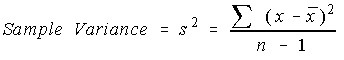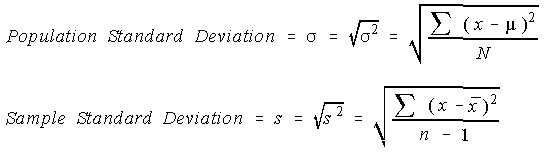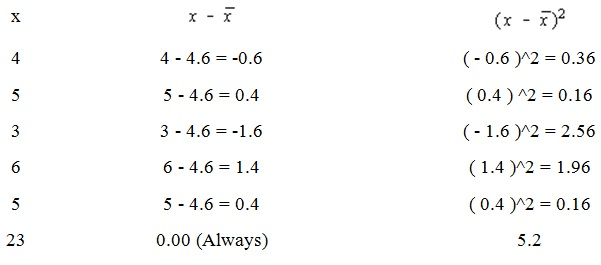#### Theory of Measures of Variation

Measures of Variation:

Range:

The simplest measure of the variation to find is termed as range. This is simply the maximum value minus the minimum value.

Range = Maximum - Minimum

It is very much affected by the extreme values as the range only uses the maximum and minimum values and it is not resistant to modify.

Variance:

Average Deviation: The range only includes the minimum and maximum numbers, and it would be advantageous to encompass a statistic that comprised all the data values.

The average deviation might be defined from the mean as:

Average deviation = Σ(x - μ)/N

The average deviation is never used because the problem is that this summation is always zero. And therefore the average deviation will always be zero.

Population Variance: To keep it from being zero, the deviation from the mean is squared and termed as the ‘squared deviation from mean’. This average squared deviation from mean is termed as the variance.

Population variance = σ2 = Σ(x - μ)2/N

Unbiased Estimate of the Population Variance:

One would predict the sample variance to be simply population variance with the population mean substituted by the sample mean. Though, one of the main uses of the statistics is to estimate the equivalent parameter. This formula has a problem which the estimated value isn't similar as the parameter. To counteract this, the sum of squares of deviations is divided by one less than the sample size.Standard Deviation:

The variances are always a problem. Keep in mind that the deviations were squared. To get the units back the similar as the original data values, the square root should be taken.The unbiased estimator for the population standard deviation is not the sample standard deviation.

The calculator doesn’t have a variance key on it. It does contain a standard deviation key. We will have to square the standard deviation to determine the variance.

Sum of Squares (shortcuts):

The sum of squares of deviations from the means is provided a shortcut notation and some alternative formulas.

SS(x) = Σ(x –x¯)2

The algebraic simplification returns:

SS(x) = Σx2 – (Σx)2/n

Now the question that comes to our mind is what is wrong with the first formula. For this let’s take an example: the last row is the totals for the columns

A) Total number of data values = 23

B) Divide by the number of values to obtain the mean: 23/5 = 4.6

C) Subtract the mean from each and every value to obtain the numbers in second column.

D) Square each and every number in the second column to obtain the values in third column.

E) Total the numbers in third column: 5.2

F) Now divide this total by one less than the sample size to obtain the variance: 5.2/4 = 1.3Not much bad, you think. However this can acquire pretty bad when the sample mean does not occur to be a ‘nice’ rational number. Think about encompassing a mean of 19/7 = 2.714285714285.... Such subtractions get horrible, and whenever you square them, they are really bad. The other problem with the first formula is that it needs to know the mean ahead of time. For a calculator, this would mean that you encompass to save all the numbers which were entered. The TI-82 does this, however most scientific calculators do not.

Now, we can examine the formula. The only things which you require to find are the sum of values and sum of the values squared. There is no subtraction, no decimals or fractions till the end. The last row includes the addition of the columns, just like previous.

a) Record each and every number in the first column and then square each number in the second column.

b) Total the first column = 23

c) Total the second column = 111

d) Calculate the sum of squares = 111 - 23*23/5 = 111 - 105.8 = 5.2

e) Divide the sum of squares by one less than the sample size to obtain the variance = 5.2/4 = 1.3

x     x2
4     16
5     25
3     9
6     36
5     25
23   111

Chebyshev's Theorem:

It states that the proportion of values which fall in k standard deviations of the mean will be at least 1 - 1 / k^2, where k is a number more than 1.

The k standard deviations understand as the interval: x bar - k * s to x bar + k * s.

No matter what the distribution is, the Chebyshev's Theorem is always true for any sample set.

Empirical Rule:

The validity of empirical rule holds true only for bell-shaped (normal) distributions.

a) Around 68% of data values fall in one standard deviation of the mean.
b) Around 95% of data values fall in two standard deviations of the mean.
c) Around 99.7% of data values fall in three standard deviations of the mean.

Using TI-82 to determine values:

We might use TI-82 to determine the measures of central tendency and the measures of variation by using the list handling capabilities of calculator.

Latest technology based Statistics Online Tutoring Assistance

Tutors, at the www.tutorsglobe.com, take pledge to provide full satisfaction and assurance in Statistics help via online tutoring. Students are getting 100% satisfaction by online tutors across the globe. Here you can get homework help for Statistics, project ideas and tutorials. We provide email based Statistics help. You can join us to ask queries 24x7 with live, experienced and qualified online tutors specialized in Statistics. Through Online Tutoring, you would be able to complete your homework or assignments at your home. Tutors at the TutorsGlobe are committed to provide the best quality online tutoring assistance for Statistics Homework help and assignment help services. They use their experience, as they have solved thousands of the Statistics assignments, which may help you to solve your complex issues of Statistics. TutorsGlobe assure for the best quality compliance to your homework. Compromise with quality is not in our dictionary. If we feel that we are not able to provide the homework help as per the deadline or given instruction by the student, we refund the money of the student without any delay.